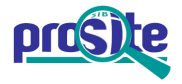Entry: PS00746

 Entry name [info] NIFH_FRXC_1 Accession [info] PS00746 Entry type [info] PATTERN Date [info] 01-DEC-1992 CREATED; 01-NOV-1995 DATA UPDATE; 02-JUN-2021 INFO UPDATE. PROSITE Doc. [info] PDOC00580

Name and characterization of the entry

 Description [info] NifH/frxC family signature 1. Pattern [info] ```E-x-G-G-P-x(2)-[GA]-x-G-C-[AG]-G. ```

Numerical results [info]

Numerical results for UniProtKB/Swiss-Prot release 2021_03 which contains 565'254 sequence entries.

 Total number of hits 231 in 231 different sequences Number of true positive hits 231 in 231 different sequences Number of 'unknown' hits 0 Number of false positive hits 0 Number of false negative sequences 1 Number of 'partial' sequences 3 Precision (true positives / (true positives + false positives)) 100.00 % Recall (true positives / (true positives + false negatives)) 99.57 %

 UniProtKB/Swiss-ProtTrue positive sequences 231 sequences ```BCHL_BRASB (A5EQ80 ), BCHL_BRASO (A4YNP4 ), BCHL_CHLAA (Q9F6X3 ), ``` ```BCHL_CHLAD (B8G943 ), BCHL_CHLCH (Q3APL1 ), BCHL_CHLL2 (B3EGV3 ), BCHL_CHLP8 (B3QQZ4 ), BCHL_CHLPB (B3ENS3 ), BCHL_CHLPD (A1BIZ7 ), BCHL_CHLPM (A4SCU6 ), BCHL_CHLSY (B9LKM2 ), BCHL_CHLT3 (B3QZE1 ), BCHL_CHLTE (Q9F714 ), BCHL_DINSH (A8LQ30 ), BCHL_HALHL (A1WXI6 ), BCHL_HELMI (B0TBM6 ), BCHL_HELMO (Q9ZGF0 ), BCHL_JANSC (Q28W37 ), BCHL_METC4 (B7KVV7 ), BCHL_METEP (A9W9E2 ), BCHL_METPB (B1ZBP2 ), BCHL_METRJ (B1LSF9 ), BCHL_METS4 (B0UDK8 ), BCHL_METSB (B8EPX5 ), BCHL_PELLD (Q3B6C6 ), BCHL_PELPB (B4SC79 ), BCHL_PROA2 (B4S591 ), BCHL_RHOCA (P0CY53 ), BCHL_RHOCB (D5ANS3 ), BCHL_RHOCS (B6ITW5 ), BCHL_RHOP2 (Q2ISY9 ), BCHL_RHOP5 (Q07RY2 ), BCHL_RHOPA (Q6N9K0 ), BCHL_RHOPB (Q219Q8 ), BCHL_RHOPS (Q132N5 ), BCHL_RHORT (Q2RWS1 ), BCHL_RHORU (Q9L8J5 ), BCHL_RHOS1 (A3PL19 ), BCHL_RHOS4 (Q9RFD6 ), BCHL_RHOS5 (A4WR94 ), BCHL_RHOSK (B9KK27 ), BCHL_ROSCS (A7NJG1 ), BCHL_ROSDO (Q16DS2 ), BCHL_ROSS1 (A5UUJ4 ), BCHL_RUBGI (Q9JPA5 ), BCHX_RHOCB (P26177 ), BCHX_RHOS4 (Q02431 ), CFBC_METAC (Q8TJZ9 ), CFBC_METBF (Q46FL1 ), CHLL_ACAM1 (B0C7T2 ), CHLL_ADICA (Q85FG5 ), CHLL_ANGEV (A2T392 ), CHLL_ANTAG (Q85A82 ), CHLL_AUXPR (Q6VQA9 ), CHLL_CHAGL (Q8LU58 ), CHLL_CHAVU (Q1ACE0 ), CHLL_CHLAT (Q19V52 ), CHLL_CHLRE (Q00469 ), CHLL_CHLVU (P56291 ), CHLL_CYAP4 (B8HUQ3 ), CHLL_CYAPA (P48110 ), CHLL_CYCTA (A6H5Q2 ), CHLL_GLOVI (Q7NI14 ), CHLL_HUPLU (Q5SCY9 ), CHLL_LARDC (Q695L6 ), CHLL_LEPBY (Q00237 ), CHLL_MARPO (P06267 ), CHLL_MESVI (Q9MUM2 ), CHLL_MICAN (B0JVB1 ), CHLL_NEPOL (Q9T399 ), CHLL_NOSP7 (B2IUL7 ), CHLL_NOSS1 (Q8YM62 ), CHLL_OLTVI (Q20EX9 ), CHLL_PHYPA (Q6YXQ7 ), CHLL_PICAB (O47041 ), CHLL_PINCO (P26181 ), CHLL_PINKO (Q85WT6 ), CHLL_PINTH (P41645 ), CHLL_PLETE (A6YGA4 ), CHLL_POLAC (P36439 ), CHLL_PORPU (P51187 ), CHLL_PROM0 (A3PBR7 ), CHLL_PROM1 (A2C0Z9 ), CHLL_PROM2 (A8G3Q8 ), CHLL_PROM3 (A2C7T4 ), CHLL_PROM4 (A9BEG6 ), CHLL_PROM5 (A2BVK5 ), CHLL_PROM9 (Q31BZ1 ), CHLL_PROMA (Q7VD39 ), CHLL_PROMM (Q7V6E7 ), CHLL_PROMP (Q7V2D7 ), CHLL_PROMS (A2BQ24 ), CHLL_PROMT (Q46GN6 ), CHLL_PYRYE (Q1XDV0 ), CHLL_RIPO1 (B7JYK3 ), CHLL_STAPU (Q32RZ7 ), CHLL_STIHE (Q06SC7 ), CHLL_SYNE7 (P54207 ), CHLL_SYNJA (Q2JS74 ), CHLL_SYNJB (Q2JMP3 ), CHLL_SYNP2 (Q53450 ), CHLL_SYNP6 (Q5N5U1 ), CHLL_SYNPW (A5GJI1 ), CHLL_SYNPX (Q7U5I2 ), CHLL_SYNR3 (A5GUB0 ), CHLL_SYNS3 (Q0I8P4 ), CHLL_SYNS9 (Q3AWT4 ), CHLL_SYNSC (Q3ALL9 ), CHLL_SYNY3 (P28373 ), CHLL_TETOB (Q1KVR9 ), CHLL_THEEB (Q8DGH0 ), CHLL_TRIEI (Q115L1 ), CHLL_TRIV2 (Q3MAN6 ), CHLL_ZYGCR (Q32RK7 ), NIFH1_AZOC5 (P26251 ), NIFH1_AZOCH (P26248 ), NIFH1_AZOVI (P00459 ), NIFH1_CLOPA (P00456 ), NIFH1_MASLA (Q47917 ), NIFH1_METBA (P54799 ), NIFH1_METIV (P51602 ), NIFH1_METTH (O27602 ), NIFH1_METTL (P25767 ), NIFH1_METTM (Q50785 ), NIFH1_NOSS1 (P00457 ), NIFH1_PAEDU (Q9AKT8 ), NIFH1_RHOCA (P0CY95 ), NIFH1_RHOCB (D5ANI3 ), NIFH1_TRIV2 (P0A3S1 ), NIFH2_AZOC5 (P26252 ), NIFH2_AZOCH (P06118 ), NIFH2_AZOVI (P15335 ), NIFH2_CLOPA (P09552 ), NIFH2_MASLA (Q47921 ), NIFH2_METBA (P54800 ), NIFH2_METIV (P08624 ), NIFH2_METTH (O26739 ), NIFH2_METTL (P08625 ), NIFH2_NOSS1 (O30577 ), NIFH2_PAEDU (Q9AKT4 ), NIFH2_RHOCA (Q07942 ), NIFH2_TRIV2 (Q44484 ), NIFH3_AZOVI (P16269 ), NIFH3_CLOPA (P09553 ), NIFH4_CLOPA (P22548 ), NIFH5_CLOPA (P09554 ), NIFH6_CLOPA (P09555 ), NIFH_ACIF2 (B7JA99 ), NIFH_ACIF5 (B5ER76 ), NIFH_ACIFR (P06661 ), NIFH_ALCFA (Q44044 ), NIFH_ALKMQ (A6TTY3 ), NIFH_ANASL (P33178 ), NIFH_AZOBR (P17303 ), NIFH_AZOPC (B6YRI6 ), NIFH_BRADU (P06117 ), NIFH_BRASP (P00463 ), NIFH_CHLCH (Q3AR70 ), NIFH_CHLL2 (B3EH88 ), NIFH_CHLP8 (B3QQ12 ), NIFH_CHLPB (B3EL81 ), NIFH_CHLPD (A1BEH0 ), NIFH_CHLPM (A4SFU6 ), NIFH_CHLTE (Q8KC92 ), NIFH_CLOAB (Q97ME5 ), NIFH_CROS5 (O07641 ), NIFH_CYAP4 (B8HWE3 ), NIFH_DECAR (Q47G67 ), NIFH_DEHM1 (Q3Z7C7 ), NIFH_DESAD (C6BX34 ), NIFH_DESAH (C0QKK8 ), NIFH_DESAL (B8FAC4 ), NIFH_DESAP (B1I0Y8 ), NIFH_DESGI (P71156 ), NIFH_DESMC (Q09158 ), NIFH_DESMR (C4XRK2 ), NIFH_DESRM (A4J8C2 ), NIFH_FRAAL (P08925 ), NIFH_FRACC (Q2J4F8 ), NIFH_FRASE (Q47922 ), NIFH_FRASN (A8L2C4 ), NIFH_FRASP (P46034 ), NIFH_GLOC7 (B7KG76 ), NIFH_GLUDA (Q9ZIE4 ), NIFH_HERSE (P77873 ), NIFH_KLEP3 (B5XPH2 ), NIFH_KLEPN (P00458 ), NIFH_LEPBY (Q00240 ), NIFH_MAGMM (A0L6X0 ), NIFH_METAR (Q0W443 ), NIFH_METHJ (Q2FUB7 ), NIFH_METJA (Q58289 ), NIFH_METMA (Q8PYY0 ), NIFH_METMI (P0CW56 ), NIFH_METMJ (A3CWW3 ), NIFH_METMP (P0CW57 ), NIFH_METNO (B8ITG7 ), NIFH_METS4 (B0UAK2 ), NIFH_METVO (P06119 ), NIFH_NOSCO (P26250 ), NIFH_NOSS6 (Q51296 ), NIFH_PECAS (Q6D2Y8 ), NIFH_PELLD (Q3B2P6 ), NIFH_PELPB (B4SC59 ), NIFH_PROA2 (B4S9H5 ), NIFH_PSEU5 (A4VJ70 ), NIFH_RHIET (P00462 ), NIFH_RHILO (Q98AP7 ), NIFH_RHILT (P00461 ), NIFH_RHIME (P00460 ), NIFH_RHOP2 (Q2J1I1 ), NIFH_RHOPS (Q13C78 ), NIFH_RHORU (P22921 ), NIFH_RHOS1 (A3PLS9 ), NIFH_RHOS4 (Q3J0H1 ), NIFH_RHOSH (O31183 ), NIFH_RIPO1 (Q55028 ), NIFH_ROSCS (A7NR80 ), NIFH_ROSS1 (A5USK5 ), NIFH_RUMCE (Q59270 ), NIFH_SINFN (P19068 ), NIFH_SYNFM (A0LH11 ), NIFH_SYNJA (Q2JTL7 ), NIFH_SYNJB (Q2JP78 ), NIFH_TERTT (C5BTB0 ), NIFH_TOLAT (C4LAS5 ), NIFH_TRIAZ (P0A3S2 ), NIFH_TRIEI (O34106 ), NIFH_TRITH (P26254 ), NIFH_WOLSU (Q7M8U8 ), NIFH_ZYMMO (Q5NLG3 ) ``` » more Retrieve an alignment of UniProtKB/Swiss-Prot true positive hits: [Clustal format, color, condensed view] [Clustal format, color] [Clustal format, plain text] [Fasta format] Retrieve the sequence logo from the alignment UniProtKB/Swiss-ProtFalse negative sequences 1 sequence ```BCHL_ACIRU (Q9WXB7 ) ``` UniProtKB/Swiss-Prot'Partial' sequences 3 sequences ```NIFH_ENTAG (P26249 ), NIFH_NOSSN (P52336 ), NIFH_RHILE (P20623 ) ``` PDB[Detailed view] 29 PDB 1CP2; 1DE0; 1FP6; 1G1M; 1G20; 1G21; 1G5P; 1M1Y; 1M34; 1N2C; 1NIP; 1RW4; » more